Chapters

Understanding mathematics means learning the language of maths in its own right.

To help you progress in the topic, here is a small lexicon of essential vocabulary to help you on your way.The best Math tutors available
1st lesson is free!4.9 (12 reviews)
Priyal
\$17
/h
1st lesson is free!4.9 (16 reviews)
Matthew
\$37
/h
1st lesson is free!5 (65 reviews)
Hamid
\$70
/h
1st lesson is free!5 (30 reviews)
Laura
\$40
/h
1st lesson is free!4.9 (18 reviews)
Alireza
\$60
/h
1st lesson is free!4.8 (10 reviews)
Stewart
\$50
/h
1st lesson is free!5 (20 reviews)
Tai
\$45
/h
1st lesson is free!5 (16 reviews)
Moos
\$45
/h
1st lesson is free!4.9 (12 reviews)
Priyal
\$17
/h
1st lesson is free!4.9 (16 reviews)
Matthew
\$37
/h
1st lesson is free!5 (65 reviews)
Hamid
\$70
/h
1st lesson is free!5 (30 reviews)
Laura
\$40
/h
1st lesson is free!4.9 (18 reviews)
Alireza
\$60
/h
1st lesson is free!4.8 (10 reviews)
Stewart
\$50
/h
1st lesson is free!5 (20 reviews)
Tai
\$45
/h
1st lesson is free!5 (16 reviews)
Moos
\$45
/h

## Learn Maths Vocabulary

To be able to get on in maths, you need to understand the instructions given.

While this may seem simple, sometimes important vocabulary is lacking.

To avoid finding yourself blocked like this, it's a good idea to revise all the important definitions, before an exam, to be able to understand and decipher the problems with which you are presented.

This will have the added benefit of making you better able to understand your maths lessons.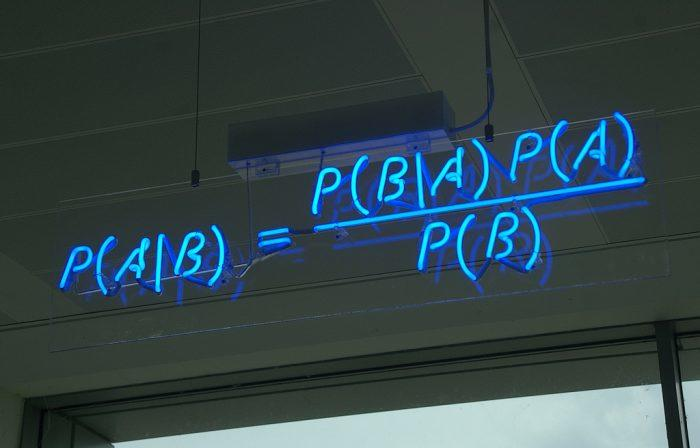Time to learn the vocabulary of maths (Source: Flickr.com - Matt Buck)

## Superprof's Mathematical Lexicon

The following dictionary of the most important definitions in maths will get you over your blockages in maths and help you to become a good student.

Become the next Albert Einstein - or even a better student - with our maths help!

### Equation

An equation is a mathematical statement containing one or more variables.

This concept is fundamental to the history of mathematics.

### Factor

A factor is an element involved in a multiplication.

In 3 x 24 = 72, 3 and 24 are the two factors.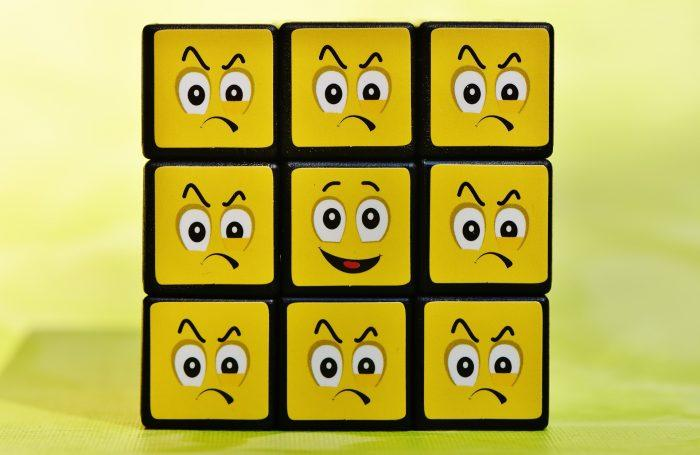The many faces of maths  (Source:  Max Pixel)

### Product

Let us take two numbers, called a and b. The product of these two numbers is the one obtained by multiplying a by b. It can also be written as x b.

### Sum

The sum is the result of adding 2 terms together. If we consider two numbers, a and b, the sum thus represents the number a, added to b, which can also be written a + b.

### Term

A "term" is each of the elements involved in a relationship, addition, subtraction, sequence, proportion or fraction.

For example: Take the sequence 1, 2, 3, 4. The 4 digits are terms. In the ratio 4/5, 4 and 5 are terms.

### Difference

In mathematics, "difference" is the result of a subtraction. 4 - 3 = 1, so here 1 is the difference.

### Dividend

When dividing two digits or numbers, the dividend is the name given to the number or number being divided. For example, in 36 ÷ 12, 36 is the dividend.

### Quotient

The quotient refers to the result obtained from a division. So, when dividing 10 by 2, the quotient is 5.

### Numerator

The numerator is the first term in a fraction. So, in the fraction 5/6, 5 is the numerator.

### Denominator

The denominator is the second term in a fraction: 6 in the fraction 5/6. It indicates in how many equivalent parts the numerator (or unit) has been divided.

### Triangle (isosceles, rectangle, equilateral)

A triangle is a three-sided polygon. An isosceles triangle is one that has two sides of the same length. A right-angled triangle is one with an angle of 90°. An equilateral triangle is a triangle whose 3 sides are the same length.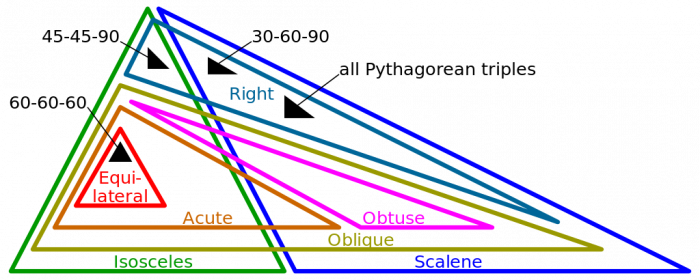Different types of triangle (Source: commons.wikimedia.org)

Do you know some of the surprising uses of maths? What about some common prejudices that people have about maths?

### Square

A square is a flat geometric figure with 4 sides are of equal length and 4 right angles (90°).

Greek mathematicians also introduced another definition of the square: the square of a number, which is the number we get after multiplying an integer by itself, so 4= 4 × 4 = 16.

### Circle

A circle is a plane curve whose set of points are equidistant from the centre point.

### Rectangle

A rectangle is a parallelogram which has two parallel opposite sides and four right (90°) angles.

A square, since it meets these two criteria, is also a rectangle.

### Diamond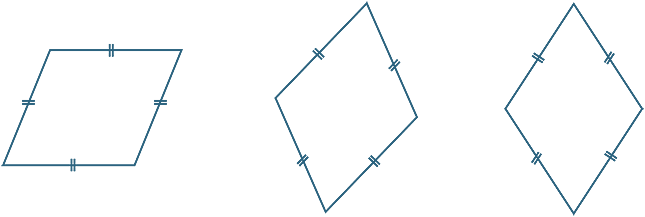The rhombus aka diamond (Source: amsi.org.au)

A diamond, also known as a rhombus, is a parallelogram whose four sides are of the same length (or 'isometric').

The opposite sides of a diamond are parallel, the opposite angles are equal, and the diagonals bisect each other to form two axes of symmetry.

### Quadrilateral

A quadrilateral is simply a four-sided polygon.

### Parallel

Parallel lines have a constant distance from each other and do not touch or intersect.

### Perpendicular

Lines are perpendicular to each other if they intersect at right angles.

### Segment

A segment is a portion of a straight line bounded by two points, which are the two ends of the segment. A segment [AB] (which is written enclosed in square brackets) has two points, A and B, as its ends. A segment can take various forms, such as:

• A circular segment, i.e. a circle
• A curved segment: A curve limited by two points.
• A segment of a right angle: A portion of a right angle, limited by 2 points.
• A portion of a straight line.

### Diagonal

In a polygon, a diagonal is a line segment that connects two non-consecutive vertices. A quadrilateral, therefore, has 2 diagonals.

### Intersection

An intersection is the meeting place of two objects: Groups or geometric objects.

You can also read about the evolution of maths in the last centuries, on our blog.

### Algebra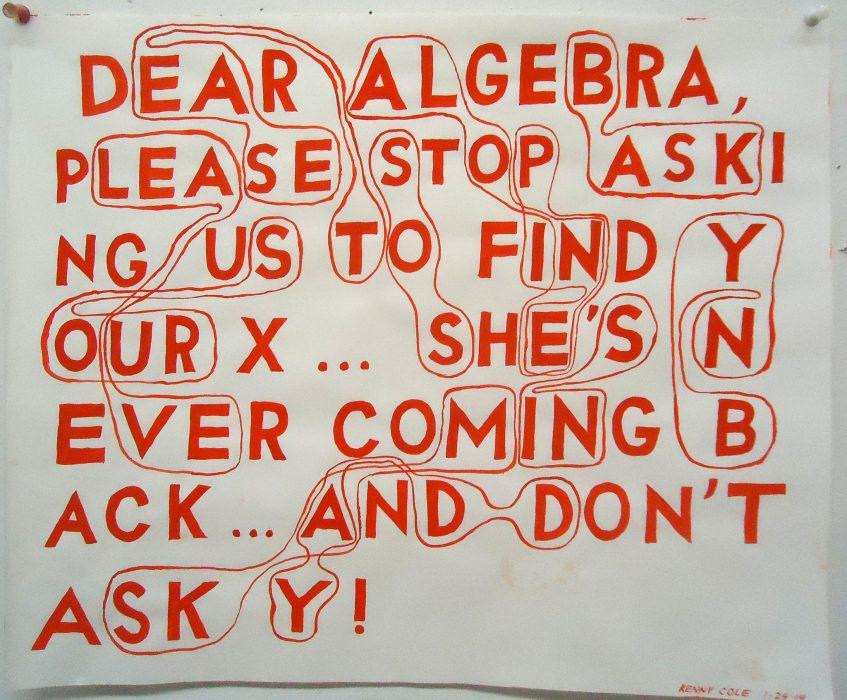Algebra can't solve everything! (Source: Flickr.com - Kenny Cole)

Algebra refers to a specific domain of mathematics which deals with calculations on the component parts of a given set of objects. Algebra is concerned with solving equations using explicit methods.

Classical algebra also deals with the theory of real numbers and complex numbers.

Algebra is an area that defines the properties of operations and how to calculate equations. Algebra can be applied be to numbers generally, as well as to geometry and complex numbers.

Searching for maths tutors is so easy on Superprof!

### Geometry

Geometry is another branch of mathematics. Its field of application extends from the study of the relations between points, curves, lines and surfaces, to the measurement of geometrical figures.

Within geometry there exist several sub-branches, including those dealing with spaces, planes, analysis and projections.

Understanding geometry also lets you understand the link between:

### Unknown

In an equation, an unknown is a missing term - by consequence, that which must be found. For example, in 5 + x = 8, x (which is equal to 3) is the unknown.

### Coordinate

To know the position of a point in two-dimensional space, you need two numbers, known as coordinates. They give the position relative to the horizontal line (abscissae) and to the vertical line (ordinates).

### Abscissa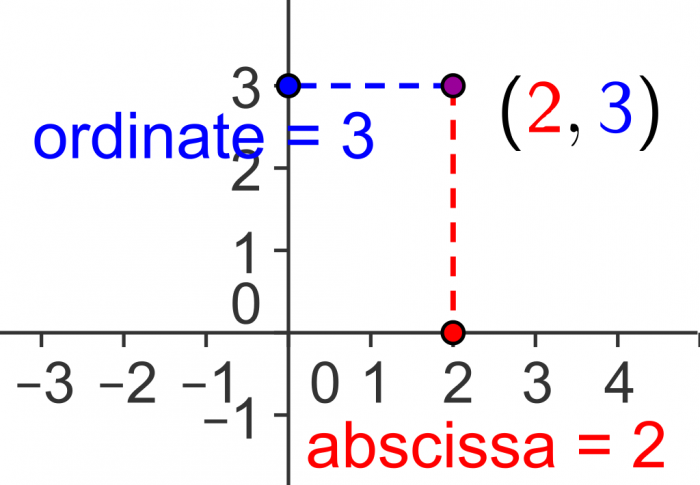An ordinate and an abscissa (Source: commons.wikimedia.org)

An abscissa is the perpendicular distance of a point from the vertical axis, and is represented by a number.

### Ordinate

As the abscissa gives the position relative to the vertical axis, the ordinate gives the position relative to the horizontal axis (up).

### Ascending and descending order

An ascending order is one that ranges from the smallest to the largest number. Conversely, a descending order is one that goes from the largest to the smallest number.

### Angle

An angle is a geometrical figure formed by two lines sharing the same point of origin. The angle is designated by a small arc, joining the two lines close to their point of origin.

Angles between 0° and 90° are known as acute and those between 90° and 180° are known as obtuse. There are many other kinds of angle, including right angles (90°), zero angles (0°), flat angles (180°) and solid angles (360°).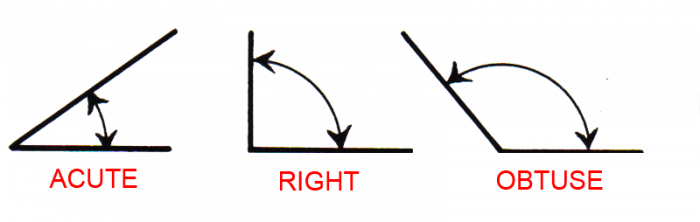Types of angle (Source: commons.wikimedia.org)

### Hypotenuse

The hypotenuse is the line opposite the right angle in a right-angled triangle.

### Graph

A graph is a visual representation consisting of points or one or more lines, of the variations in a measurable quantity.

### Theorem

A theorem is a demonstrable theory which results from other propositions which have already been demonstrated. Pythagoras' and Thales' theorems are among the most widely-known and quoted.

If you have any questions about the origins of maths or mathematical misconceptions, make sure to ask for help in your private maths lessons.

Need help mastering these concepts? Search for an online math tutor.

Need a Math teacher?

Enjoyed this article?5.00/5 - 1 review(s)Loading...Jon

As an Englishman in Paris, I enjoy growing my knowledge of other languages and cultures. I'm interested in History, Economics, and Sociology and believe in the importance of continuous learning.